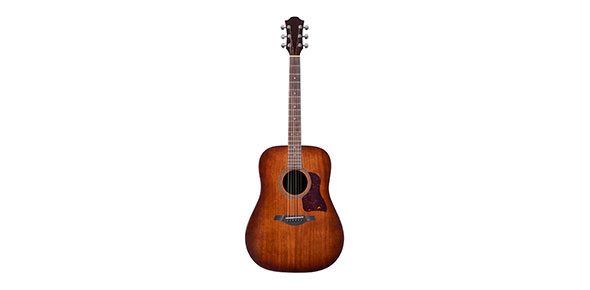# Music 101 Final Review

25 Questions | Total Attempts: 80SettingsA review of terminology from weeks 1 through 13

• 1.
Which type of scale(s) contains a leading tone?
• A.

Major & natural minor

• B.

Major & harmonic minor

• C.

None of these

• 2.
The smallest distance between any two pitches is
• A.

A half step

• B.

A whole step

• C.

Neither of these

• 3.
What notes are included in a major triad whose root is C?
• A.

C, E, G

• B.

C, E-flat, G

• C.

C, E, G-sharp

• D.

C, E-flat, G-flat

• 4.
The note that is the foundation of a chord / triad is called the
• A.

Root

• B.

Tonic

• C.

Keynote

• D.

None of these

• 5.
The first note of a scale, the most central and important note of the scale, is called the
• A.

Tonic/keynote

• B.

• C.

Root

• D.

None of these

• 6.
A P4 above F is
• A.

B

• B.

B-flat

• C.

B-sharp

• D.

None of these

• 7.
A M3 above G is
• A.

B

• B.

B-flat

• C.

A-sharp

• D.

None of these

• 8.
A minor 6 above D is
• A.

B

• B.

B-flat

• C.

A

• D.

A-flat

• 9.
A minor 3 above A is
• A.

C

• B.

C-sharp

• C.

C-flat

• D.

None of these

• 10.
A M7 above B is
• A.

A-sharp

• B.

A

• C.

B-flat

• 11.
A P5 above B-flat is
• A.

F

• B.

F-sharp

• C.

E

• D.

E-flat

• 12.
What notes are in an a harmonic minor scale?
• A.

A, b, c, d, e, f, g-sharp, a

• B.

A, b, c, d, e, f, g, a

• C.

A, b, c, d, e, f-sharp, g-sharp, a

• D.

A, b, c-sharp, d, e, f-sharp, g-sharp, a

• 13.
What notes are in a B-flat major scale?
• A.

B-flat, C, D, E-flat, F, G, A, B-flat

• B.

B-flat, C, D, E-flat, F, G, A, B

• C.

B-flat, C, D, E-flat, F, G, A-flat, B-flat

• D.

B-flat, C, D, E, F, G, A, B-flat

• 14.
What notes are in a g natural minor scale?
• A.

G, a, b-flat, c, d, e-flat, f, g

• B.

G, a, b, c, d, e-flat, f, g

• C.

G, a, b-flat, c, d, e-flat, f-sharp, g

• D.

G, a, b, c, d, e, f-sharp, g

• 15.
The relative minor for the key of F-sharp major is
• A.

D-sharp minor

• B.

D minor

• C.

E-flat minor

• D.

None of these

• 16.
The relative minor for the key of B-flat major is
• A.

G minor

• B.

G-flat minor

• C.

G-sharp minor

• 17.
A major key and its relative minor share
• A.

A tonic

• B.

A key signature

• C.

A key note

• D.

None of these

• 18.
The distance between two notes played in succession is called a
• A.

Melodic interval

• B.

Harmonic interval

• C.

Neither of these

• 19.
The distance between two notes played simultaneously is called a
• A.

Melodic interval

• B.

Harmonic interval

• C.

Neither of these

• 20.
What is the significance of the word "fifths" in the "circle of fifths"?
• A.

There are only five keys in the circle

• B.

Each key is the interval of a P5 from each adjacent key

• C.

Only keys with five sharps or flats are included

• 21.
What key has six sharps in its key signature?
• A.

F-sharp major/d-sharp minor

• B.

F major/d minor

• C.

Neither of these

• 22.
What key has seven flat in its key signature?
• A.

C-flat major / a-flat minor

• B.

C major / a minor

• C.

G-flat major / e-flat minor

• D.

None of these

• 23.
What key has 5 sharps in its key signature?
• A.

B major / g-sharp minor

• B.

C-sharp major / a-sharp minor

• C.

F-sharp major / d-sharp minor

• 24.
What key has no sharps or flats in its key signature?
• A.

C major / a minor

• B.

G major / e minor

• C.

F major / d minor

• 25.
What is the meter for the time signature: 12/16?
• A.

• B.

Compound triple

• C.

Compound duple

• D.Back to top